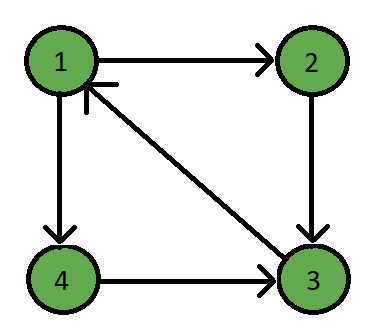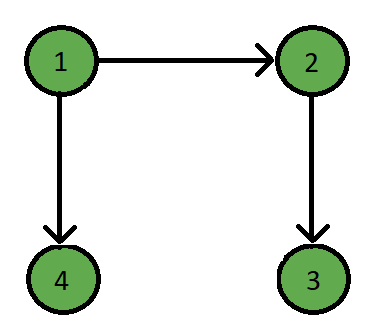GeeksforGeeks App
Open AppBrowser
Continue

# Java Program to Find a Good Feedback Edge Set in a Graph

Feedback edge set is a set of edges where F ⊆ E of a directed graph G, whose every cycle must contain at least one edge from F.

In simple words, Feedback edge set is a set of edges whose removal from the graph makes the graph directed acyclic graph.

Examples:

Input:Output:

Feedback Edge Set: ( 3 -> 1 ) ( 4 -> 3 )

Explanation:Clearly two edges 3 -> 1 and 4 -> 3 will make the graph acyclic.

Approach:

A feedback edge set can be find out with simple BFS but if the given graph is a DAG then there have to be no edge set.

1. Check if the given graph is already a directed acyclic graph and remove all the sink vertex.
2. Return the modified graph and run BFS.
3. Mark the visited vertices while running BFS.
4. If marked vertex is visited once again, then print out that edge as feedback edge.

Code:

## Java

 `// Java Program to find a good feedback``// edge set in a graph` `import` `java.util.*;`` ` `class` `Graph``{``    ``// Map for storing graph in adj list``    ``private` `Map> adjacencyList;` `    ``// Graph Constructor``    ``public` `Graph(``int` `v)``    ``{``        ``// Create adj List``        ``adjacencyList = ``new` `HashMap>();``        ` `        ``// Create empty adj list for each vertex``        ``for` `(``int` `i = ``1``; i <= v; i++)``        ``{``            ``adjacencyList.put(i, ``new` `LinkedList());``        ``}``    ``}` `    ``// Adding new edge``    ``public` `void` `setEdge(``int` `src, ``int` `dest)``    ``{``        ``List neighbours = adjacencyList.get(src);``        ``neighbours.add(dest);``    ``}`` ` `    ``// Function for checking DAG``    ``// and removing sink vertex``    ``public` `Graph checkAcyclic()``    ``{``        ``Integer count = ``0``;``        ` `        ``// Iterator for all the vertices``        ``Iterator nodes  = ``this``.adjacencyList.keySet().iterator();``        ``Integer size = ``this``.adjacencyList.size() - ``1``;``        ` `        ``// Traverse till the last node``        ``while` `(nodes.hasNext())``        ``{``            ``Integer i = nodes.next();``            ` `            ``// Get the neighbours of the selected vertex``            ``List adjList = ``this``.adjacencyList.get(i);``            ` `            ``// If the given graph is DAG``            ``if` `(count == size)``            ``{``                ``return` `this``;``            ``}``            ` `            ``// If it's a sink vertex``            ``if` `(adjList.size() == ``0``)``            ``{``                ``count++;``                ``Iterator neighbour``                  ``= ``this``.adjacencyList.keySet().iterator();``                ` `                ``// Remove all edges from that vertex``                ``while` `(neighbour.hasNext())``                ``{``                    ``Integer j = neighbour.next();``                    ``List edges  = ``this``.adjacencyList.get(j);``                  ` `                    ``if` `(edges.contains(i))``                    ``{``                        ``edges.remove(i);``                    ``}``                ``}``                ` `                ``// Remove the vertex from the graph``                ``this``.adjacencyList.remove(i);``              ` `                ``nodes = ``this``.adjacencyList.keySet().iterator();``            ``}``        ``}``        ``// Return the modified graph``        ``return` `this``;``    ``}`` ` `    ``// Function to find the``    ``// feedback edge set``    ``public` `boolean` `getFeedbackEdgeSet()``    ``{``        ``int` `v=``this``.adjacencyList.size();``        ``boolean` `flag = ``false``;``        ` `        ``// Array to mark the visited vertices``        ``int``[] visited = ``new` `int``[v + ``1``];``        ` `        ``// Iterator for all the vertices``        ``Iterator nodes``          ``= ``this``.adjacencyList.keySet().iterator();``        ` `        ``// Traverse till the last node``        ``while` `(nodes.hasNext())``        ``{``            ``Integer i = nodes.next();``            ` `            ``// Get the neighbours of the vertex``            ``List neighbours = ``this``.adjacencyList.get(i);``          ` `            ``visited[i] = ``1``;``            ``if` `(neighbours.size() != ``0``)``            ``{``                ``for` `(``int` `j = ``0``; j < neighbours.size(); j++)``                ``{``                    ``// If the vertex is already visited``                    ``if` `(visited[neighbours.get(j)] == ``1``)``                    ``{``                        ``// Mark flag to true denoting``                        ``// the given graph is not DAG``                        ``flag = ``true``;``                      ` `                        ``System.out.print(``"( "``+i+``" -> "``+``                                         ``neighbours.get(j)+``" ) "``);``                    ``}``                    ` `                    ``// Mark if not visited yet``                    ``else``                    ``{``                        ``visited[neighbours.get(j)] = ``1``;``                    ``}``                ``}``            ``}``        ``}``        ``return` `flag;``    ``}``}` `// Driver Code``public` `class` `GFG``{``    ``public` `static` `void` `main(String args[])``    ``{``        ``// Number of vertices and edges``        ``int` `v = ``4``;``        ``int` `e = ``5``;``        ` `        ``// Initialize new Graph``        ``Graph g = ``new` `Graph(v);``        ` `        ``// Edges``        ``g.setEdge(``1``,``2``);``        ``g.setEdge(``2``,``3``);``        ``g.setEdge(``4``,``3``);``        ``g.setEdge(``1``,``4``);``        ``g.setEdge(``3``,``1``);``        ` `        ``// Run the function``        ``g = g.checkAcyclic();``      ` `        ``System.out.print(``"Feedback Edge Set: "``);``      ` `        ``if` `(g.getFeedbackEdgeSet() == ``false``)``        ``{``            ``System.out.println(``"None"``);``        ``}``    ``}``}`

Output

`Feedback Edge Set: ( 3 -> 1 ) ( 4 -> 3 ) `

Time Complexity: O(E*V) where E is the number of edges and V is the number of vertices.

My Personal Notes arrow_drop_up